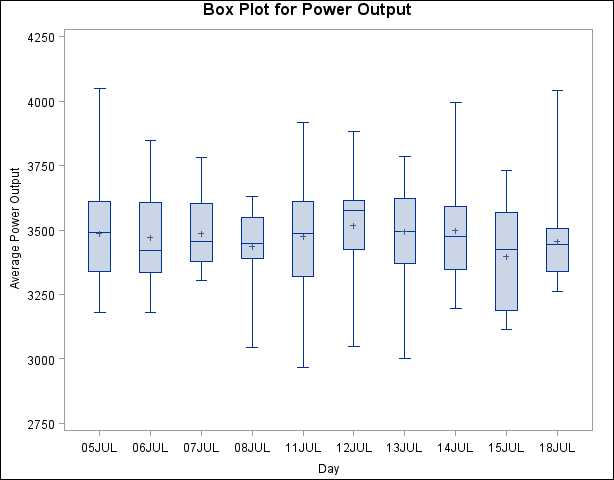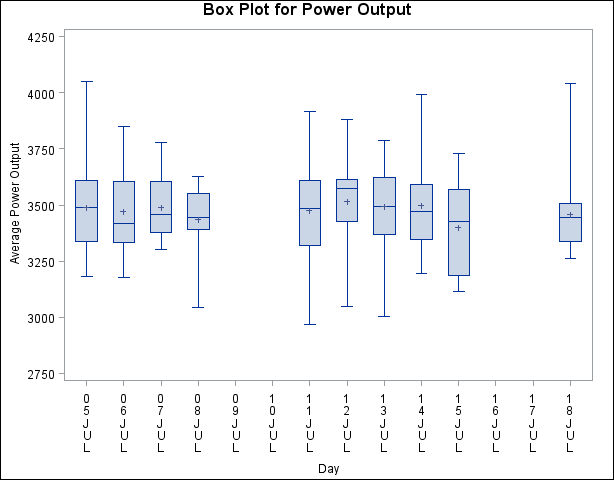Continuous Group Variables

By default, the PLOT statement treats numerical group variable values as discrete values and spaces the boxes evenly on the plot. The following statements produce the box plot in Figure 25.9:

```ods graphics off;
title 'Box Plot for Power Output';
proc boxplot data=Turbine;
plot KWatts*Day;
run;
```

Figure 25.9 Box Plot with Discrete Group VariableThe labels on the horizontal axis in Figure 25.9 do not represent 10 consecutive days, but the box-and-whiskers plots are evenly spaced.

In order to treat the group variable as continuous, you can specify the CONTINUOUS or HAXIS= option when producing traditional graphics. Either option produces a box plot with a horizontal axis scaled for continuous group variable values. (ODS Graphics does not support a continuous group axis.)

The following statements produce the plot shown in Figure 25.10. The TURNHLABELS option orients the horizontal axis labels vertically so there is room to display them all.

```title 'Box Plot for Power Output';
proc boxplot data=Turbine;
plot KWatts*Day / turnhlabels
continuous;
run;
```

Figure 25.10 Box Plot with Continuous Group VariableNote that the tick values on the horizontal axis represent consecutive days and that no box-and-whiskers plots are displayed for days when no turbine data were collected.